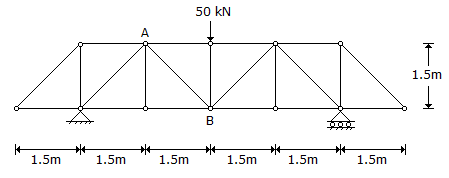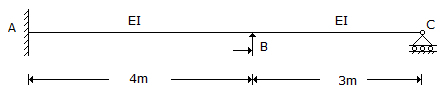# Civil Engineering - UPSC Civil Service Exam Questions

16.

Consider the following statements :
1. A recovery ratio of less than 1 implies that the soil has compressed.
2. A recovery ratio greater than 1 implies that the soil has swelled.
3. A recovery ratio of less than 1 implies that the soil has swelled.
4. A recovery ratio greater than 1 implies that the soil has compressed.
Which of the following statements given above is/are correct ?

 A. 1 and 2 B. 1 only C. 3 and 4 D. 4 only

Explanation:

No answer description available for this question. Let us discuss.

17.

The force in the member 'AB' of the truss shown in the given figure isA. 25 kN compressive B. 25 2 tensile C. 25 2kN compressive D. 25 kN tensile

Explanation:

No answer description available for this question. Let us discuss.

18.

What are the distribution factors at joint B for the members BA and BC respectively, in the figure at side ?A. 0.57 and 0.43 B. 0.43 and 0.57 C. 0.50 and 0.50 D. 0.36 and 0.64

Explanation:

No answer description available for this question. Let us discuss.

19.

If the gradient of a ground is -3%, that of another ground intersecting with the former is 5%, and the horizontal length of the vertical curve to be drawn is 600 m, then the vertical offset 'e' from the vertex of the vertical curve to the mid-point of the curve will be

 A. 1.5 m B. 6 m C. 15 m D. 75 m

Explanation:

No answer description available for this question. Let us discuss.

20.

Why is super plasticizer added to concrete?
1. To reduce the quantity of mixing water.
2. To increase the consistency.
3. To reduce the quantity of cement.
4. To increase resistance to freezing and thawing.
Select the correct answer using the code given below :

 A. 1, 2 and 3 B. 1, 3 and 4 C. 2 and 3 D. 4 only